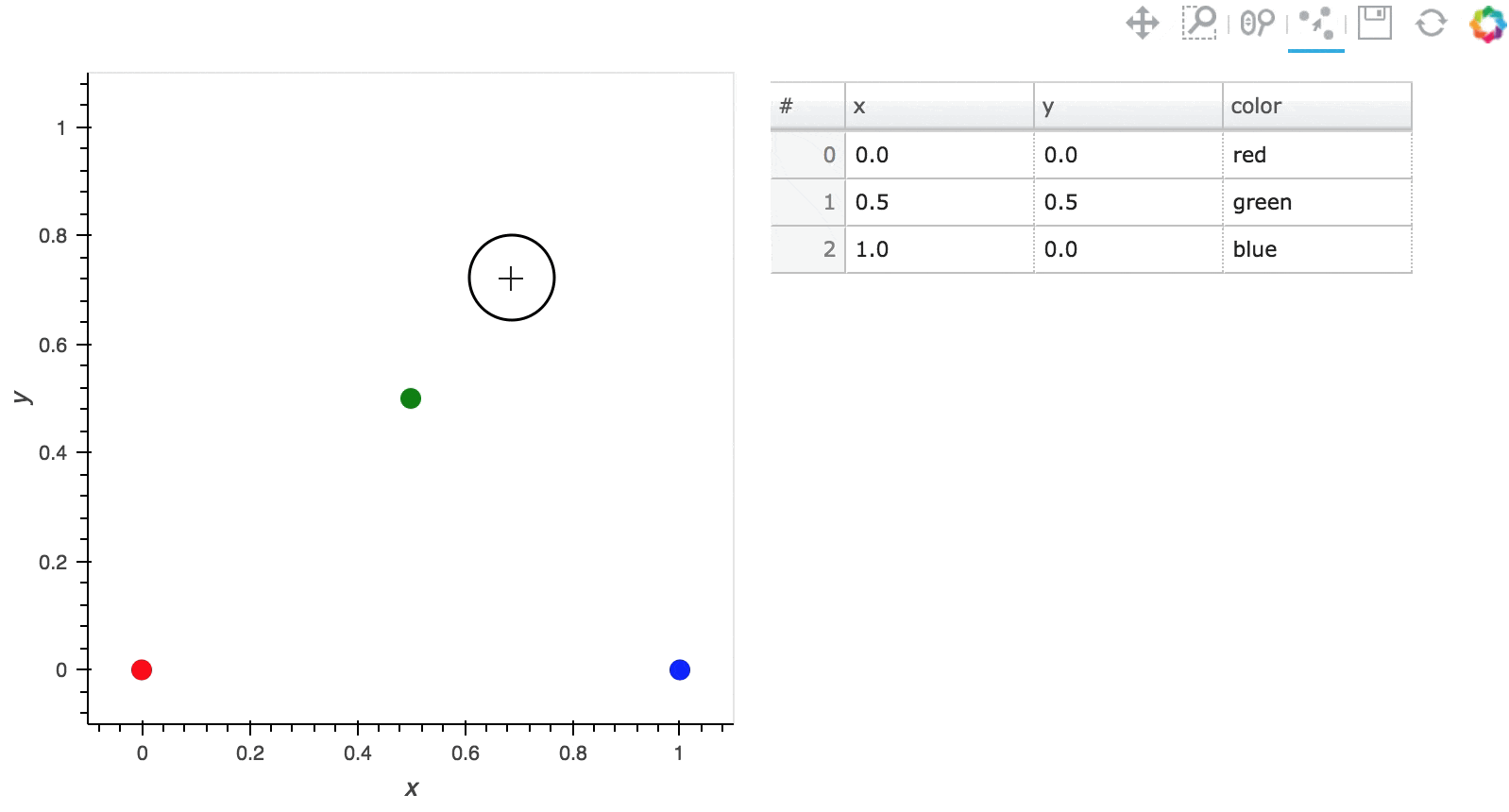# PointDraw#

## Title: PointDraw Stream#

Description: A linked streams example demonstrating how to use the PointDraw stream.

Dependencies: Bokeh

Backends: Bokeh

```import holoviews as hv
from holoviews import opts, streams

hv.extension('bokeh')
```The `PointDraw` stream adds a bokeh tool to the source plot, which allows drawing, dragging and deleting points and making the drawn data available to Python. The tool supports the following actions:

```Tap anywhere on the plot
```

Move point

```Tap and drag an existing point, the point will be dropped once you let go of the mouse button.
```

Delete point

```Tap a point to select it then press BACKSPACE or DELETE key while the mouse is within the plot area.
```

As a simple example we will create a `PointDraw` stream and attach it to a set of `Points` with a color dimension. By using a `DataLink` we can additionally link the `Points` to a Table containing the same data.

We can now add drag and delete points, see the x/y position change in the table and edit the a color for each point in the table. Additionally the `empty_value` parameter on the `PointDraw` stream lets us define the value that will be inserted on columns other than the x/y position, which we can use here to set new points to ‘black’. Finally we can limit the number of points using the `num_objects` option, ensuring that once the limit is reached the oldest point is dropped.

```data = ([0, 0.5, 1], [0, 0.5, 0], ['red', 'green', 'blue'])
points = hv.Points(data, vdims='color').redim.range(x=(-.1, 1.1), y=(-.1, 1.1))
point_stream = streams.PointDraw(data=points.columns(), num_objects=10, source=points, empty_value='black')
table = hv.Table(points, ['x', 'y'], 'color')

(points + table).opts(
opts.Layout(merge_tools=False),
opts.Points(active_tools=['point_draw'], color='color', height=400,
size=10, tools=['hover'], width=400),
opts.Table(editable=True))
```Whenever the data source is edited the data is synced with Python, both in the notebook and when deployed on the bokeh server. The data is made available as a dictionary of columns:

```point_stream.data
```
```{'x': [0.0, 0.5, 1.0],
'y': [0.0, 0.5, 0.0],
'color': array(['red', 'green', 'blue'], dtype='<U5')}
```

Alternatively we can use the `element` property to get an Element containing the returned data:

```point_stream.element
```
This web page was generated from a Jupyter notebook and not all interactivity will work on this website. Right click to download and run locally for full Python-backed interactivity.